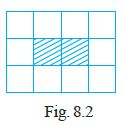#### Fill in the blanks to make the statement true.Use Fig. 8.2 (In which each square is of unit length) for questions 39 and 40:The ratio of the area of the shaded portion to that of the whole figure is ______.Area of shaded portion =1 x 2= 2 square units

Area of whole figure =3 x 4 =12 square units

The ratio of the area of the shaded portion to that of the whole figure is